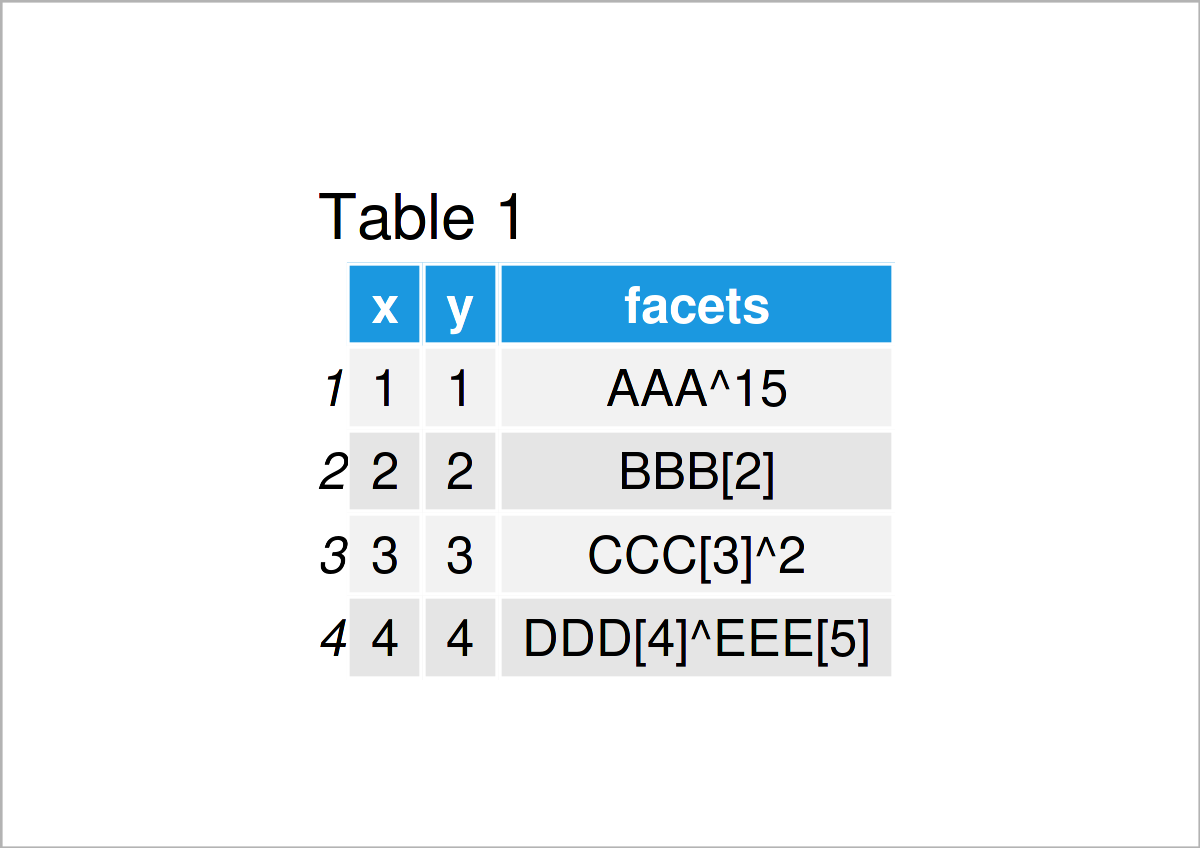# Add Subscript & Superscript to Labels of ggplot2 Facet Plot in R (Example)

In this R tutorial you’ll learn how to draw labels with subscripts and superscripts in a ggplot2 facet plot.

The tutorial will contain this content:

So without further additions, let’s just jump right in.

## Exemplifying Data, Add-On Packages & Basic Plot

Consider the exemplifying data below:

```data <- data.frame(x = 1:4,        # Create example data frame
y = 1:4,
facets =  c("AAA^15",
"BBB",
"CCC^2",
"DDD^EEE"))
data                               # Print example data frame```Table 1 shows the structure of our example data: It consists of four rows and three columns.

In order to draw our data with the ggplot2 package, we also need to install and load ggplot2 to RStudio:

```install.packages("ggplot2")        # Install & load ggplot2
library("ggplot2")```

As a next step, we can plot our data in a facet plot using the facet_wrap function:

```ggplot(data, aes(x, y)) +          # Draw facet plot without subscript/superscript
geom_point() +
facet_wrap(facets ~ .)```By executing the previous R syntax, we have created Figure 1, i.e. a facet plot. As you can see, this facet plot does not contain any subscripts and superscripts yet.

## Example: Add Subscripts & Superscripts to Labels of ggplot2 Facet Plot Using labeller Argument

The following code explains how to add labels to a facet plot that contain subscripts and superscripts.

To accomplish this, we have to set the labeller argument within the facet_wrap function to be equal to label_parsed:

```ggplot(data, aes(x, y)) +          # Draw facet plot with subscript/superscript
geom_point() +
facet_wrap(facets ~ .,
labeller = label_parsed)```By executing the previous syntax, we have created Figure 2, i.e. a new facet plot where the labels contain subscripts and superscripts.

## Video, Further Resources & Summary

In case you need further explanations on the R codes of this page, I recommend having a look at the following video on my YouTube channel. In the video, I’m explaining the R programming code of this article in a live session.

Please accept YouTube cookies to play this video. By accepting you will be accessing content from YouTube, a service provided by an external third party.If you accept this notice, your choice will be saved and the page will refresh.

Also, you could have a look at some of the other articles on this website:

In this post, I have explained how to add labels with subscripts and superscripts in a ggplot2 facet graphic in R programming. If you have additional comments or questions, please let me know in the comments section below. Furthermore, don’t forget to subscribe to my email newsletter in order to get updates on new posts.

Subscribe to the Statistics Globe Newsletter